# PLL Ideal Behavior

A PLL will adjust the phase of its output such that its reference input REFclk and its feedback clock are perfectly aligned, or in phase.

Consider the ASIC PLL circuit shown in Figure C.2. In this ideal circuit, the PLL will perfectly align the arrival time of the feedback clock, t FB , with the arrival time of the reference clock, t REF (t FB = t REF ). The output of the PLL is distributed throughout the ASIC with the use of a clock-distribution network that is perfectly balanced such that the delay from the PLL output to every ASIC register is equal (dly a = dly b ).

##### Figure C.2. Ideal PLL Behavior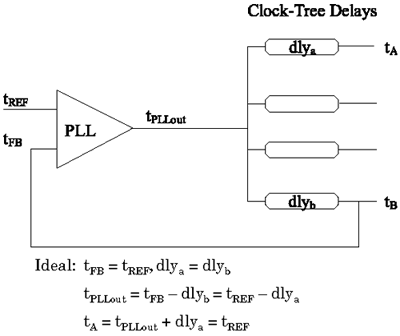The arrival time of the PLL output can be expressed as:

Equation C.1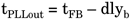Since in this ideal case t REF = t FB and dly a = dly b ,

Equation C.2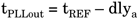or

Equation C.3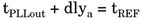and the arrival time of the clock at a register, regA, can be expressed as

Equation C.4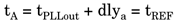So, in this case, the arrival time of a clock at any register is perfectly aligned with t REF . Note that the delay of the clock-distribution network is not a term of the arrival time, t A . This shows how a PLL can be used to minimize the effects of on-chip clock latency.

Consider also two ASICs fed by the same clock, but whose clock-distribution networks have different latency times. Using PLLs in each of the ASICs will make the respective clocks appear to be in phase with each other.

Equation C.5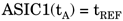and

Equation C.6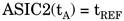hence,

Equation C.7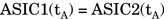Top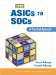From ASICs to SOCs: A Practical Approach
ISBN: 0130338575
EAN: 2147483647
Year: 2003
Pages: 61

Similar book on Amazon

flylib.com © 2008-2017.
If you may any questions please contact us: flylib@qtcs.net